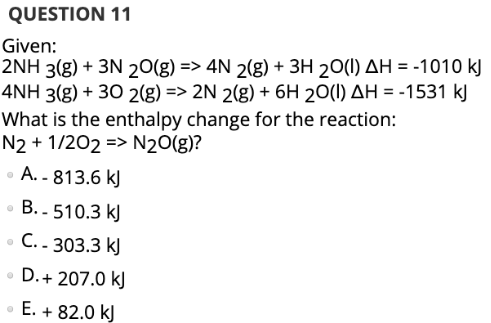# Given: 2NH 3(g) + 3N 2O(g) => 4N 2(g) + 3H 2O(l) ΔH = -1010 kJ 4NH 3(g) + 3O 2(g) => 2N 2(g) + 6H 2O(l) ΔH = -1531 kJ What is the enthalpy change for the reaction : N2 + 1/2O2 => n2O(g)? A. -813.6 kJ B. -510.3 kJ C. -303.3 kJ D. +207.0 kJ E. +82.0 kJ